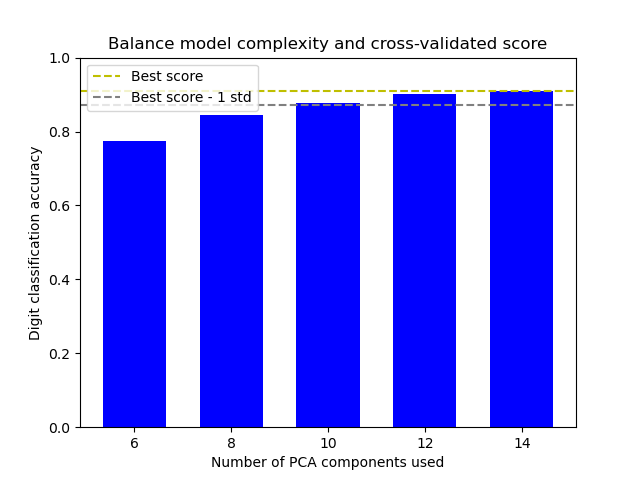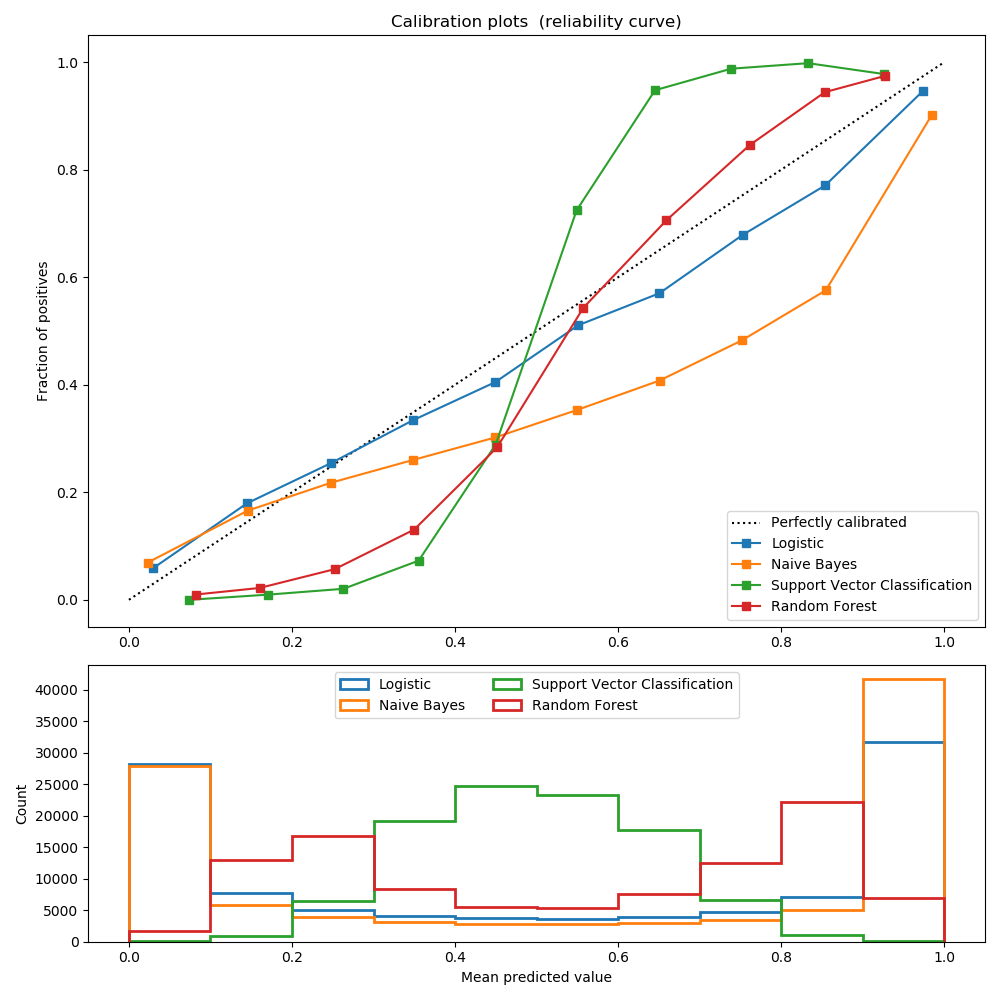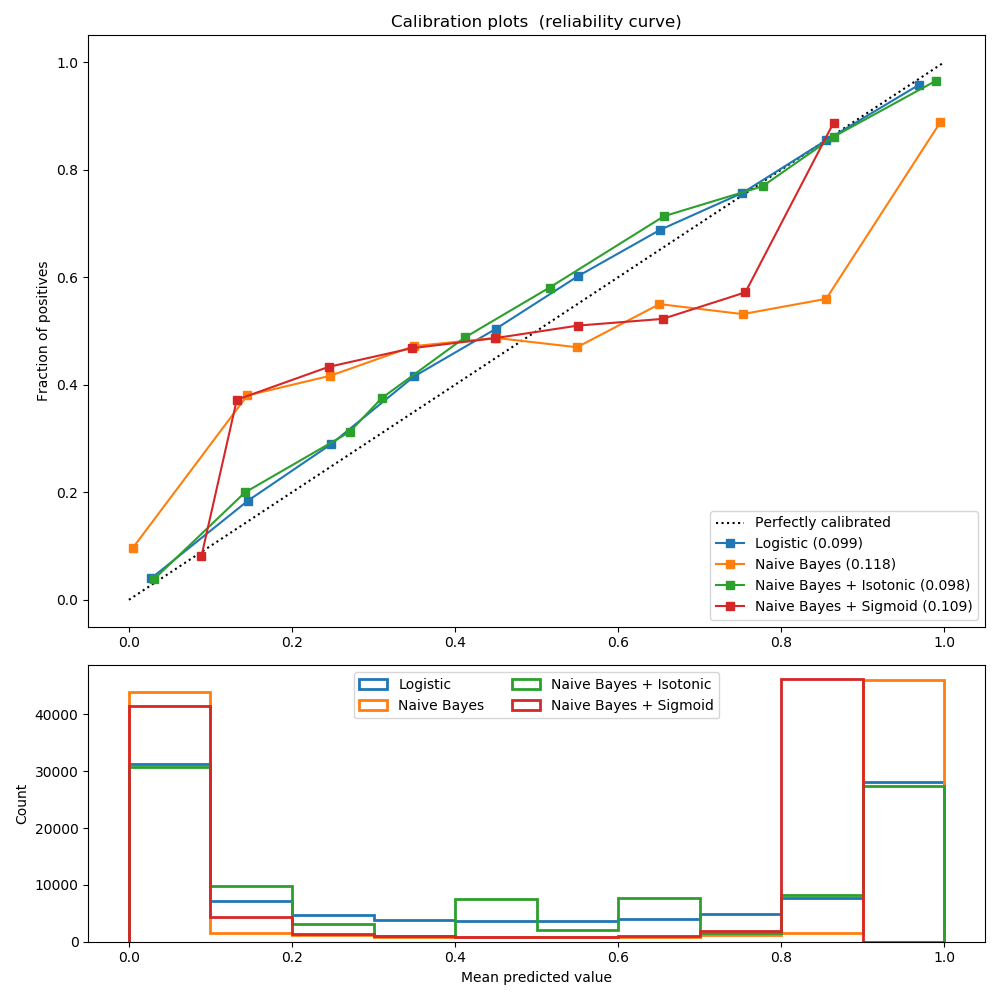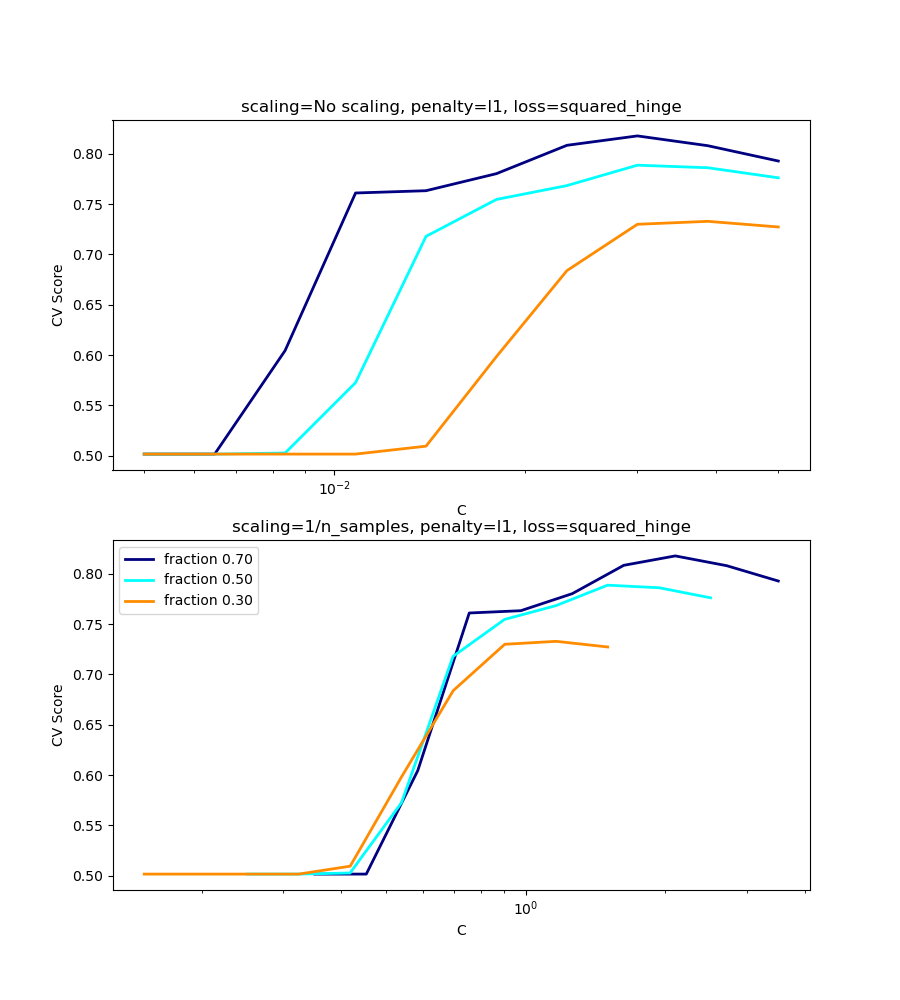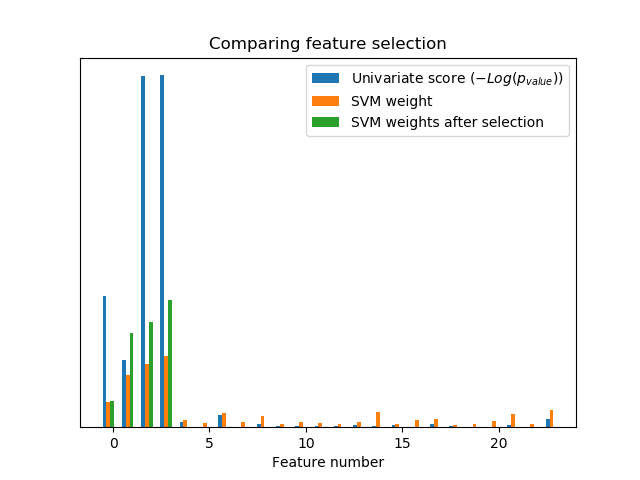sklearn.svm.LinearSVC¶

class sklearn.svm.LinearSVC(penalty='l2', loss='squared_hinge', *, dual=True, tol=0.0001, C=1.0, multi_class='ovr', fit_intercept=True, intercept_scaling=1, class_weight=None, verbose=0, random_state=None, max_iter=1000)

penalty {‘l1’, ‘l2’}, default=’l2’

loss {‘hinge’, ‘squared_hinge’}, default=’squared_hinge’

dual bool, default=True

tol float, default=1e-4

C float, default=1.0

multi_class {‘ovr’, ‘crammer_singer’}, default=’ovr’

fit_intercept bool, default=True

intercept_scaling float, default=1

class_weight dict or ‘balanced’, default=None

verbose int, default=0

random_state int or RandomState instance, default=None

max_iter int, default=1000

coef_ ndarray of shape (1, n_features) if n_classes == 2 else (n_classes, n_features)

intercept_ ndarray of shape (1,) if n_classes == 2 else (n_classes,)

classes_ ndarray of shape (n_classes,)

n_iter_ int

SVC

sklearn.linear_model.SGDClassifier

SGDClassifier可以通过调整损失和损失参数来优化与LinearSVC相同的成本函数。 另外，它需要更少的内存，允许增量（在线）学习，并实现各种损失函数和正则化机制。

LIBLINEAR: A Library for Large Linear Classification

>>>

>>> from sklearn.svm import LinearSVC
>>> from sklearn.pipeline import make_pipeline
>>> from sklearn.preprocessing import StandardScaler
>>> from sklearn.datasets import make_classification
>>> X, y = make_classification(n_features=4, random_state=0)
>>> clf = make_pipeline(StandardScaler(),
...                     LinearSVC(random_state=0, tol=1e-5))
>>> clf.fit(X, y)
Pipeline(steps=[('standardscaler', StandardScaler()),
('linearsvc', LinearSVC(random_state=0, tol=1e-05

>>>

>>> print(clf.named_steps['linearsvc'].coef_)
[[0.141...   0.526... 0.679... 0.493...]]

>>>

>>> print(clf.named_steps['linearsvc'].intercept_)
[0.1693...]
>>> print(clf.predict([]))


decision_function(self,X) 预测样本的置信度得分。
densify(self) 将系数矩阵转换为数组格式。
fit(self, X, y[, sample_weight]) 根据给定的训练数据拟合模型。
get_params(self[, deep]) 获取这个估计器的参数。
predict(self, X) 预测X中样本的类别标签。
score(self, X, y[, sample_weight]) 返回给定测试数据和标签上的平均准确度。
set_params(self, **params) 设置这个估计器的参数。
sparsify(self) 将系数矩阵转换为稀疏格式。
__init__(self, penalty='l2', loss='squared_hinge', *, dual=True, tol=0.0001, C=1.0, multi_class='ovr', fit_intercept=True, intercept_scaling=1, class_weight=None, verbose=0, random_state=None, max_iter=1000)

decision_function(self, X)

X array_like or sparse matrix, shape (n_samples, n_features)

result array, shape=(n_samples,) if n_classes == 2 else (n_samples, n_classes)

densify(self)

self Fitted estimator
fit(self, X, y, sample_weight=None)

X {array-like, sparse matrix} of shape (n_samples, n_features)

y array-like of shape (n_samples,)

sample_weight array-like of shape (n_samples,), default=None

self object

get_params(self, deep=True)

deep bool, default=True

params mapping of string to any

predict(self, X)

X array_like or sparse matrix, shape (n_samples, n_features)

C array, shape [n_samples]

score(self, X, y, sample_weight=None)

X array-like of shape (n_samples, n_features)

y array-like of shape (n_samples,) or (n_samples, n_outputs)
X的真实标签
sample_weight array-like of shape (n_samples,), default=None

score float

set_params(self, **params)

**params dict

self object

sparsify(self)

Intercept_成员未转换。

self 拟合估计量

sklearn.svm.LinearSVC使用示例¶